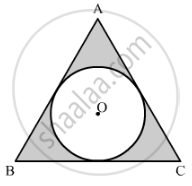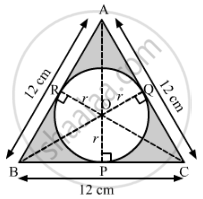# In  Fig 4, a circle is inscribed in an equilateral triangle ABC of side 12 cm. Find the radius of inscribed circle and the area of the shaded region. - Mathematics

In  Fig 4, a circle is inscribed in an equilateral triangle ABC of side 12 cm. Find the radius of inscribed circle and the area of the shaded region.[Use π=3.14 and √3=1.73]#### Solution

It is given that ABC is an equilateral triangle of side 12 cm.
Construction:
Join OA, OB and OC.

Draw:
OPBC
OQAC
ORABLet the radius of the circle be r cm.
Area of ∆AOB + Area of ∆BOC + Area of ∆AOC = Area of ∆ABC

1/2xxABxxOR+1/2xxBCxxOP+1/2xxACxxOQ=sqrt3/4xx(side)^2

1/2xx12xxr+1/2xx12xxr+1/2xx12xxr=sqrt3/4xx(12)^2

3xx1/2xx12xxr=sqrt3/4xx12xx12

r=2sqrt3=2xx1.73=3.46

Therefore, the radius of the inscribed circle is 3.46 cm.

Now, area of the shaded region = Area of ∆ABC − Area of the inscribed circle

=[sqrt3/4xx(12)^2-pi(2sqrt3)^2] cm^2

=[36sqrt3-12pi]cm^2

=[36xx1.73-12xx3.14] cm^2

=[62.28-37.68]cm^2

=24.6 cm2

Therefore, the area of the shaded region is 24.6 cm2

Concept: Circumference of a Circle
Is there an error in this question or solution?